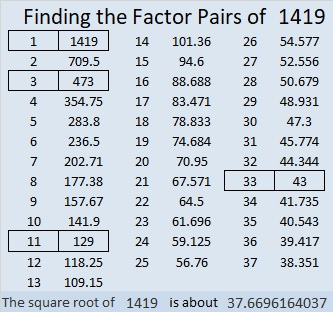# 1419 and Level 1

This Level 1 Find the Factors 1-12 puzzle is as simple as clockwork. Can you find all the factors?Print the puzzles or type the solution in this excel file: 12 Factors 1419-1429

Now I’ll tell you a few things about the puzzle number, 1419:

• 1419 is a composite number.
• Prime factorization: 1419 = 3 × 11 × 43.
• 1419 has no exponents greater than 1 in its prime factorization, so √1419 cannot be simplified.
• The exponents in the prime factorization are 1, 1, and 1. Adding one to each exponent and multiplying we get (1 + 1)(1 + 1)(1 + 1) = 2 × 2 × 2 = 8. Therefore 1419 has exactly 8 factors.
• The factors of 1419 are outlined with their factor pair partners in the graphic below.1419 is the difference of two squares in four different ways:
710² – 709² = 1419
238² – 235² = 1419
70² – 59² = 1419
38² – 5² = 1419

That last one means we are only 25 numbers away from the next perfect square, and that seems like clockwork, too.

1419 is only the second Zeisel number: (105 was the first.)
(4×1-1)(4×3-1)(4×11-1) = 3×11×43 =1419

This site uses Akismet to reduce spam. Learn how your comment data is processed.